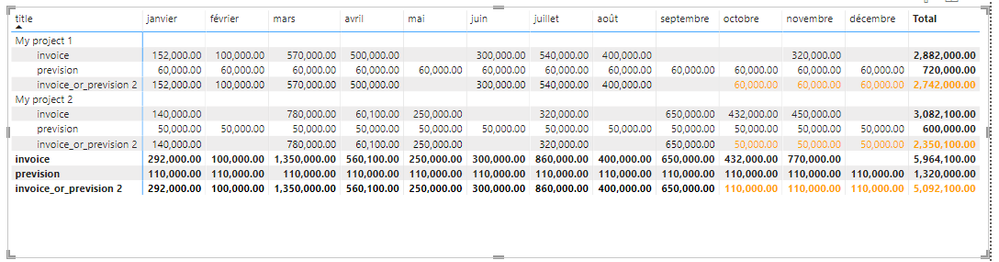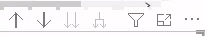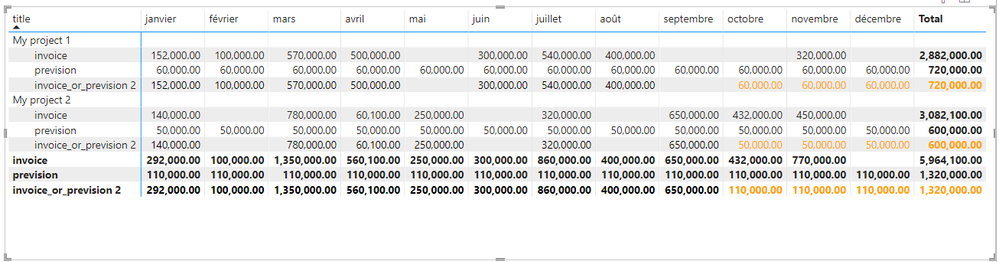cancel
Showing results for
Did you mean:Frequent Visitor

## Conditional value inside matrix table

Hi all,

I'm trying to display a conditional value in a matrix according to the column.

I made a small example. You can find the file here : https://drive.google.com/file/d/13pp0m-LjFgYZIWyEXAm49tP-BtnQnkyf/view?usp=sharing

The file has 4 tables :

1. invoice : representing the sales invoices
2. prevision : representing the turnovers that we expect to make in the future
3. project : representing the projects on which we work
4. date : a calculated date table

Each invoice and prevision are related to a project.
Each invoice and prevision are related to the date table.

Each month, we want to analyse the last month and discuss about the previsions of each project.

For that, we have a parameter called "Cut off date" which is the separation between the past and the future.

I made a matrix table where i want to display one line per project with as value :

• the total invoice amount of the month if month is before the cut off date
• the total prevision amount of the month if month is after the cut off date

To do that I used the following DAX :

``````invoice_or_prevision = CALCULATE(
IF(
FIRSTNONBLANK('date'[before_cut_off_date], TRUE()),
SUM(invoice[amount]),
SUM(prevision[amount])
)
)``````

It works for each month column but not for the total (and it's logical)

Any idea of how I can achieve this ?

Thanks,

Vincent

1 ACCEPTED SOLUTIONCommunity Support

I have corrected the measure. Please check it in the new pbix.

``````invoice_or_prevision 2 =
VAR _maxDate = [max_date]
RETURN
IF (
ISINSCOPE ( 'date'[Month] ),
IF (
MAX ( 'date'[Date] ) < _maxDate,
SUM ( invoice[amount] ),
SUM ( prevision[amount] )
),
CALCULATE ( SUM ( invoice[amount] ), ALLSELECTED ( 'date'[Date] ), 'date'[Date] < _maxDate )
+ CALCULATE ( SUM ( prevision[amount] ), ALLSELECTED ( 'date'[Date] ), 'date'[Date] >= _maxDate )
)
``````Best Regards,
Community Support Team _ Jing
If this post helps, please Accept it as Solution to help other members find it.

4 REPLIES 4Frequent Visitor

I just needed to change it a little bit for my real file :

• Use 'date'[Date].[Mois] ('date'[Date].[Month] in english) in the ISINSCOPE because I wanted to keep the real date as column (It allows to navigate between years with the following arrows)
• Replace "ALLSELECTED" with "VALUES" because I have a segment on 'date'[Date] and want to apply it to the matrix. That way, the sum will only take into account the filtered dates

The final code (for my case) :

``````invoice_or_prevision 2 =
VAR _maxDate = [max_date]
RETURN
IF (
ISINSCOPE ( 'date'[Date].[Mois] ),
IF (
MAX ( 'date'[Date] ) < _maxDate,
SUM ( invoice[amount] ),
SUM ( prevision[amount] )
),
CALCULATE ( SUM ( invoice[amount] ), ALLSELECTED ( 'date'[Date] ), 'date'[Date] < _maxDate )
+ CALCULATE ( SUM ( prevision[amount] ), ALLSELECTED ( 'date'[Date] ), 'date'[Date] >= _maxDate )
)``````

Thanks a lot !Community Support

Not sure if I understand your expected output correctly. I made several changes in your example file and get the following result. Is this what you want?Main changes are:

1. Expand the range of Date table;

2. Create a new "invoice_or_prevision 2" measure;

3. Create a color measure for conditional formatting.

Let me know if this doesn't meet your requirement. I have attached the file at bottom.

Best Regards,
Community Support Team _ Jing
If this post helps, please Accept it as Solution to help other members find it.Frequent Visitor

Hi, @v-jingzhang ,

1. You're right, in my main file, I also expanded the range of the date table (my main file is more complicated)
2. your measure "invoice_or_prevision 2" is cleaner than mine
3. your measure "Color Measure" is cleaner than my "color" measure.

But, it is not 100% correct. There is still a problem with the totals :The total displayed is not correct : the sum of the first 3 columns are greater than 720k.

In fact, the displayed total is the total of the previsions, not the total of the invoices until the "cut off date" + the total of the previsions from the "cut off date".

I think this is because the condition in "invoice_or_prevision" (yours or mine) measure is called for all the months at same time (and not per month)

Thank you,

Best regards,Community Support

I have corrected the measure. Please check it in the new pbix.

``````invoice_or_prevision 2 =
VAR _maxDate = [max_date]
RETURN
IF (
ISINSCOPE ( 'date'[Month] ),
IF (
MAX ( 'date'[Date] ) < _maxDate,
SUM ( invoice[amount] ),
SUM ( prevision[amount] )
),
CALCULATE ( SUM ( invoice[amount] ), ALLSELECTED ( 'date'[Date] ), 'date'[Date] < _maxDate )
+ CALCULATE ( SUM ( prevision[amount] ), ALLSELECTED ( 'date'[Date] ), 'date'[Date] >= _maxDate )
)
``````Best Regards,
Community Support Team _ Jing
If this post helps, please Accept it as Solution to help other members find it.